## Python爬取201865条《隐秘的角落》弹幕，发现看剧不如爬山？ 转

o
osc_4yp05nbl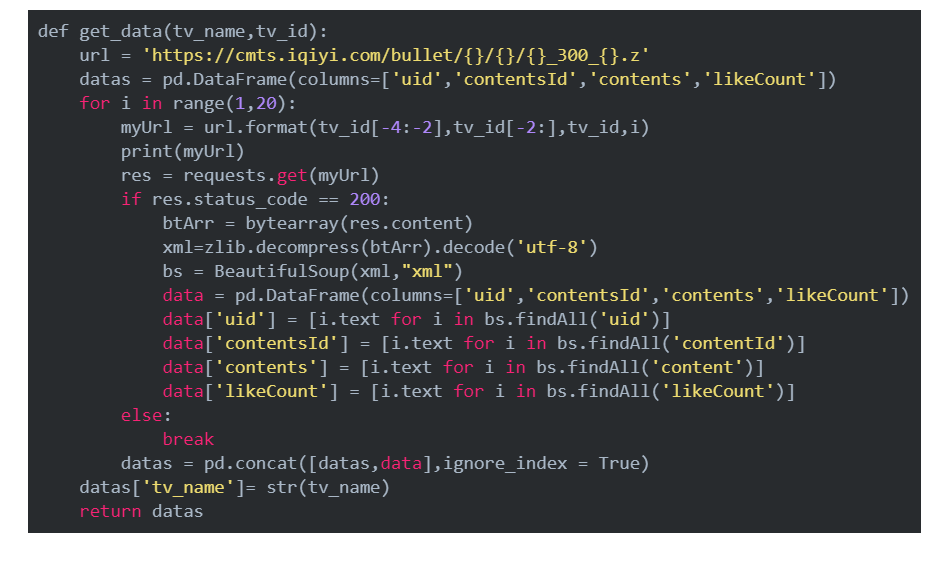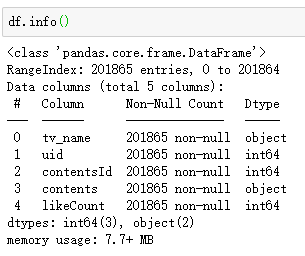# 累计发送弹幕数的用户

danmu_counts = df.groupby('uid')['contentsId'].count().sort_values(ascending = False).reset_index()
danmu_counts.columns = ['用户id','累计发送弹幕数']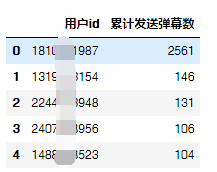df_top1 = df[df['uid'] == 1810351987].sort_values(by="likeCount",ascending = False).reset_index()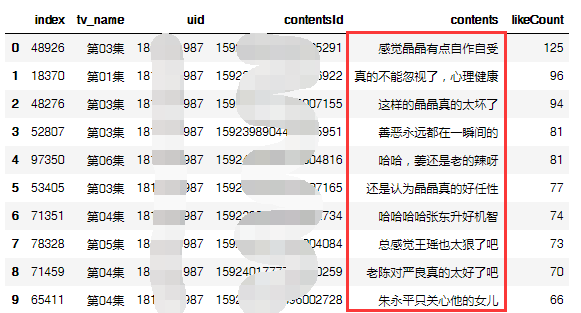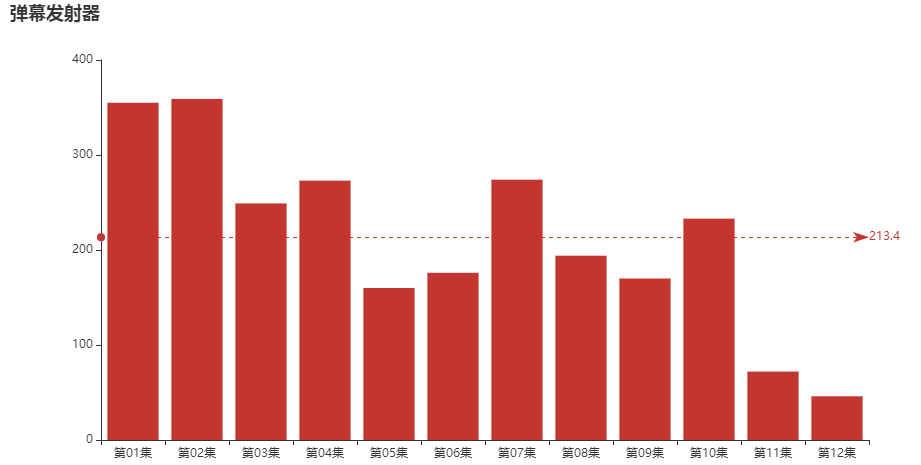“弹幕发射器”同志，11、12集请加大输出！

df_like = df[df.groupby(['tv_name'])['likeCount'].rank(method="first", ascending=False)==1].reset_index()[['tv_name','contents','likeCount']]
df_like.columns = ['剧集','弹幕','赞']
df_like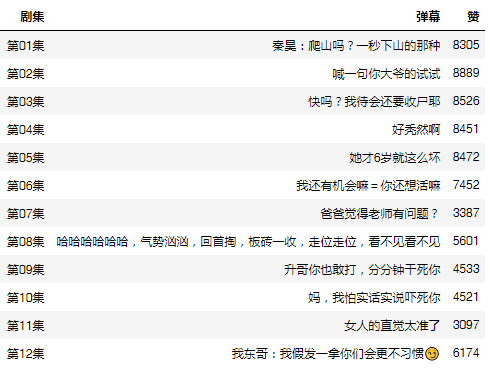a = {'张东升':'东升|秦昊|张老师', '朱朝阳':'朝阳', '严良':'严良', '普普':'普普', '朱永平':'朱永平', '周春红':'春红|大娘子', '王瑶':'王瑶', '徐静':'徐静|黄米依', '陈冠声':'王景春|老陈|陈冠声', '叶军':'叶军|皮卡皮卡', '马主任':'主任|老马', '朱晶晶':'晶晶','叶驰敏':'叶驰敏'}

a = {'张东升':'东升|秦昊|张老师', '朱朝阳':'朝阳', '严良':'严良', '普普':'普普', '朱永平':'朱永平', '周春红':'春红|大娘子', '王瑶':'王瑶', '徐静':'徐静|黄米依', '陈冠声':'王景春|老陈|陈冠声', '叶军':'叶军|皮卡皮卡', '马主任':'主任|老马', '朱晶晶':'晶晶','叶驰敏':'叶驰敏'}
for key, value in a.items():

``df[key] = df['contents'].str.contains(value)``

staff_count = pd.Series({key: df.loc[df[key], 'contentsId'].count() for key in a.keys()}).sort_values()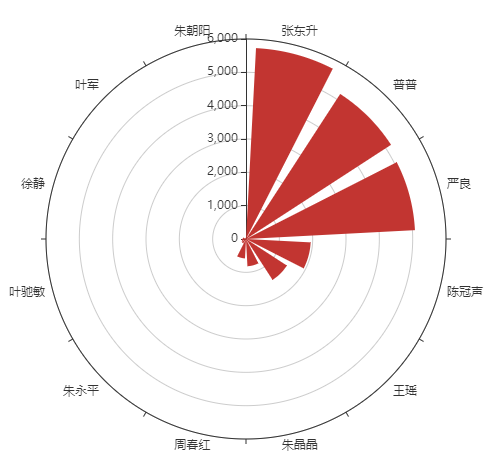import stylecloud
from IPython.display import Image

stylecloud.gen_stylecloud(text=' '.join(text1), collocations=False,

``````                      font_path=r'‪C:\Windows\Fonts\msyh.ttc',
icon_name='fas fa-play-circle',size=400,
output_name='隐秘的角落-词云.png')``````

Image(filename='隐秘的角落-词云.png')20万条弹幕词云https://alltodata.cowtransfer.com/s/5b483c08987243

【云栖号在线课堂】每天都有产品技术专家分享！

【云栖号在线课堂 社群】https://c.tb.cn/F3.Z8gvnK

o

### osc_4yp05nbl#### 暂无文章

19分钟前
6
0

fyin1314
27分钟前
14
0

38分钟前
6
0
Spring Boot + Spring Security自定义用户认证

12
0
DateTime2与SQL Server中的DateTime - DateTime2 vs DateTime in SQL Server

13
0